# 机器学习算法之_决策树（DecisionTree）

【DecisionTree】

### 一、原理

##### 2.找对象的故事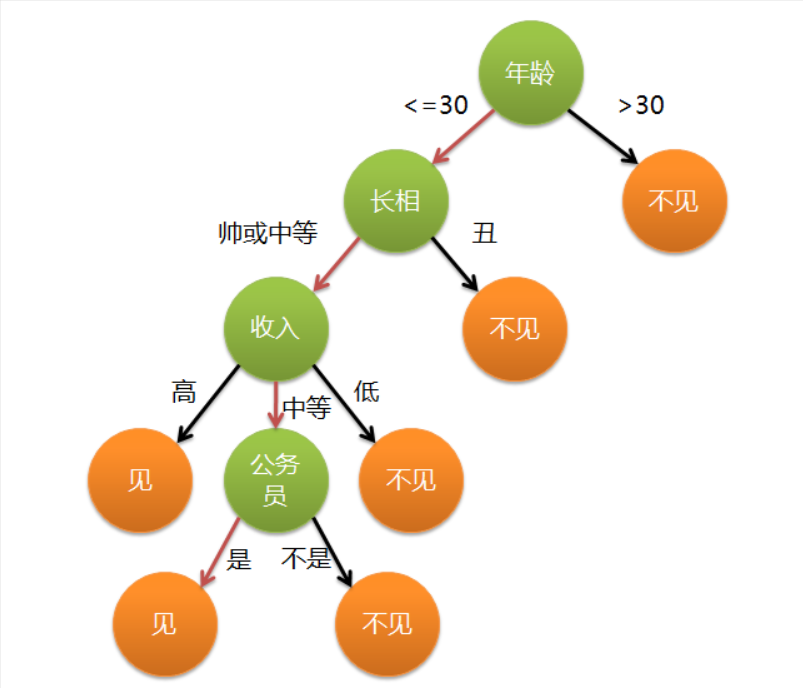### 三、决策树的构造

##### 2.分裂属性

（1）属性是离散值且不要求生成二叉决策树。此时用属性的每一个划分作为一个分支。

（2）属性是离散值且要求生成二叉决策树。此时使用属性划分的一个子集进行测试，按照“属于此子集”和“不属于此子集”分成两个分支。

（3）属性是连续值。此时确定一个值作为分裂点split_point，按照>split_point和<=split_point生成两个分支。

##### 3.ID3算法

entropy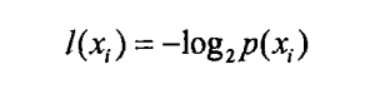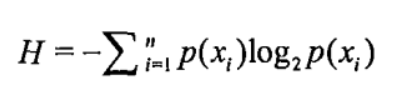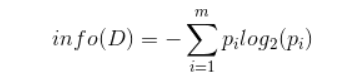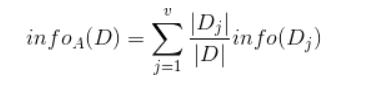ID3算法就是在每次需要分裂时，计算每个属性的增益率，然后选择增益率最大的属性进行分裂。下面我们继续用SNS社区中不真实账号检测的例子说明如何使用ID3算法构造决策树。为了简单起见，我们假设训练集合包含10个元素：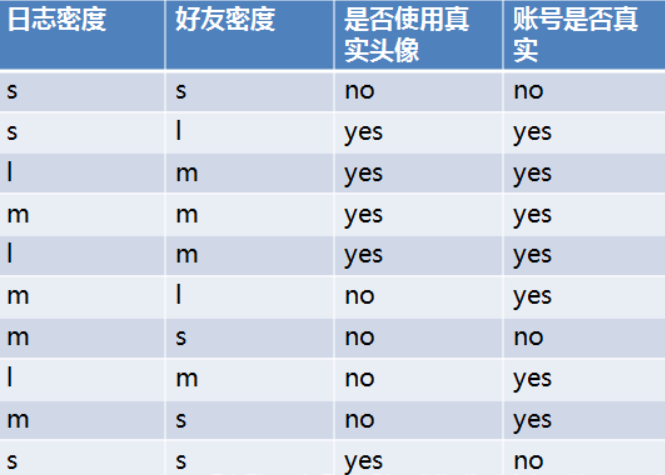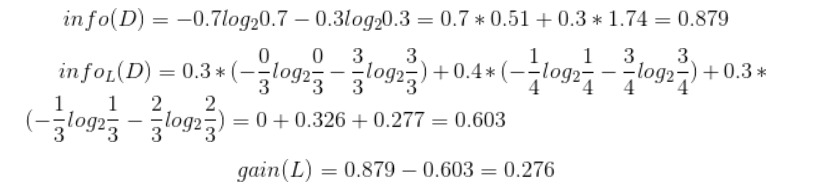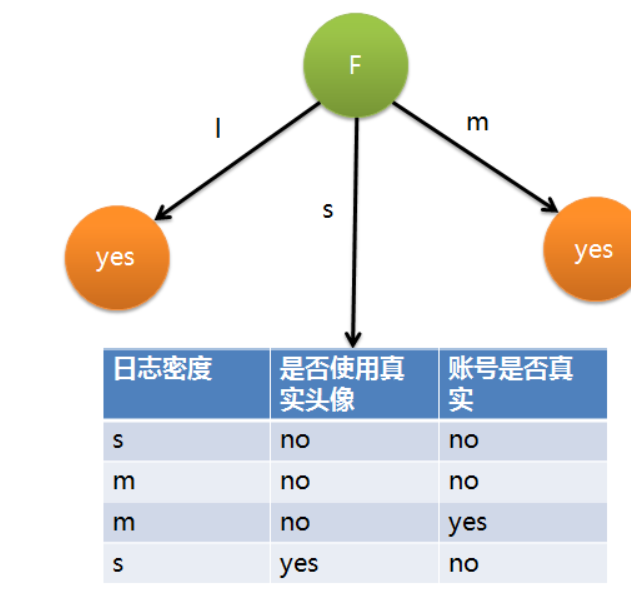【练习】计算上图的信息熵，确定下一个分类的特征

#先求目标值的熵
import numpy as np
#根据账户是否真实求一下熵，
#求解账户是否真实的信息熵
#0.3 no    0.7 yes
info_D = -(0.3*np.log2(0.3) + 0.7*np.log2(0.7))
info_D

#日志密度对账户是否真实的期望信息有多大
#日志密度 L
#L分类：s:0.3--->1/3yes  2/3no
#m:0.4========>1/4no    3/4yes
#l:0.3====>1/1yes
info_L_D = -(0.3*(1/3*np.log2(1/3)+ 2/3*np.log2(2/3))+
0.4*(3/4*np.log2(3/4) + 1/4*np.log2(1/4))+
0.3*1*np.log2(1))
info_L_D

#日志密度的信息增益
info_D - info_L_D

#查看一下好友密度的信息增益
#s:0.4----->1/4yes  3/4no
#m:0.4------>1/1
#l:0.2======>1/1
info_F_D = -(0.4*(1/4*np.log2(1/4) + 3/4*np.log2(3/4)))
info_F_D

#是好友密度的信息增益
info_D - info_F_D



### 四、实战

【注意】 参数max_depth越大，越容易过拟合

##### 1、使用自带的iris数据集
# 使用决策树算法，导包
from sklearn.tree import DecisionTreeClassifier
from sklearn.neighbors import KNeighborsClassifier
from sklearn.linear_model import LogisticRegression
import sklearn.datasets as datasets

data  = iris.data
target = iris.target

from sklearn.model_selection import train_test_split
X_train,X_test,y_train,y_test = train_test_split(data, target, test_size = 0.2)

dt = DecisionTreeClassifier(max_depth=100)
dt.fit(X_train,y_train)

#特征的重要性
dt.feature_importances_

dt.score(X_test,y_test)


knn = KNeighborsClassifier()
knn.fit(X_train,y_train)
knn.score(X_test, y_test)


logic = LogisticRegression()
logic.fit(X_train,y_train)
logic.score(X_test, y_test)


### 2.使用回归算法预测一个椭圆

#使用决策树的回归问题
from sklearn.tree import DecisionTreeRegressor
import pandas  as pd
from pandas import Series,DataFrame
import matplotlib.pyplot as plt
%matplotlib inline

#角度值, 训练样本
x = np.linspace(-100,100, 100)

#x1 和y1 组成一个点？，并且这个点 在圆上面, 这一个点就是一个目标值
#sin(x)^2 + cos(x)^2 = 1   x^2 + y^2 = 1
x1 = np.sin(x)
y1 = np.cos(x)

plt.scatter(x1, y1)
plt.axis("equal")

#对目标值进行添加噪声
y1[::5] += np.random.randn(20)*0.2

plt.scatter(x1, y1)
plt.axis('equal')


#生成测试数据
X_test = np.linspace(-100,100,1000).reshape(1000,1)
dt1 = DecisionTreeRegressor(max_depth=1000)
dt1.fit(x.reshape(100,1), np.c_[x1, y1])
#预测出来圆上面的点
y1_ = dt1.predict(X_test)
y1_

Series(y1_[:,0]).unique()


plt.scatter(y1_[:,0], y1_[:,1], c = "red")
plt.scatter(x1, y1, alpha = 0.2)
plt.axis("equal")


https://blog.csdn.net/xo3ylAF9kGs/article/details/78623265或百度了解

©️2019 CSDN 皮肤主题: 书香水墨 设计师: CSDN官方博客